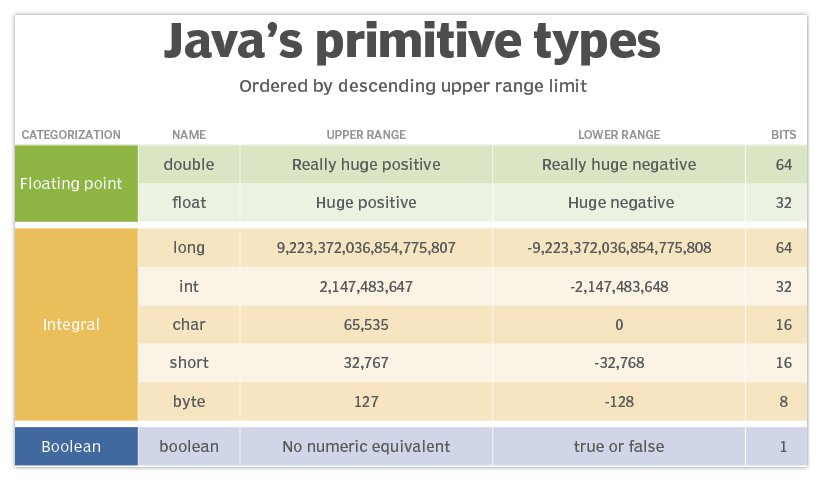# Integer vs. int: What's the difference?

## Difference between int and Integer

The key difference between the Java int and Integer types is that an int simply represents a whole number, while an Integer has additional properties and methods. The int is one of Java’s eight Java primitive types, while the Integer wrapper class is one of hundreds of components included in the Java API.

When you declare a variable of type int in Java, that variable represents the number assigned to it, and that’s all.

• You can’t call a method on an int.
• You can’t access any properties of an int.
• You can’t compare an int with the .equals method.
• You can’t extend an int through inheritance.
• The Object class is not an ancestor of the int.

An int simply represents the numeric value assigned to it. You can’t get any more information out of an int other than the whole number it represents.

### How do you initialize an int in Java?

All you can do with an int is access its value so that you can use it in mathematical and comparison operations.

```int x = 10;  // declare an int named x with a value of 10
int y = 20;  // declare an int named y with a value of 20
int sum = x + y;  // perform addition with a Java int
int difference = x-y;  // perform subtraction with a Java int
boolean flag = (x == y);  // compare two int values```

## Java Integer and int type comparison

In contrast to the primitive type int, Integer is a full-blown Java class. This creates a long list of difference, such as:

1. The Integer class is an Object while an int is a primitive type.
2. The Integer is compared with .equals while the int uses two equal signs, == .
3. The Integer class is a wrapper class for the int, while the int is not a class at all.
4. The Integer class allows conversion to float, double, long and short, while the int doesn’t.
5. The Integer consumes slightly more memory than the 32-bit Java int.
6. The Integer class has five static properties while the int can’t have any.
7. The Integer class has two constructors while the int can’t have any.
8. The Integer class has over 30 static methods, while the int can’t have any.
9. The Integer class has over a dozen instance methods, while the int can’t have any.
10. You can convert text Strings to int values with Integer, but you can’t with the int.

The purpose of the Integer class is to keep track of the value of a single int, so the Integer class holds onto a single int value internally. This is why it is often called a wrapper class.

## How to create an Integer vs. int in Java

While there is really only one way to initialize an int directly, you can initialize Integer types in Java multiple ways, including:

• Through a constructor.
• From the value of a String.
• With the help of autoboxing.

Here are three examples of how to declare and initialize an Integer vs an int in Java:

```Integer number = new Integer(10); // constructor
Integer dozen = Integer.valueOf("20"); // convert String to int
Integer century = 100; // autoboxing

// compare the initialization of int vs Integer types
int kilo = 1000;
Integer kilometer = new Integer(1000);```Unlike the Integer, int is one of Java’s 8 primitive type. Integer is a reference type.

## Java Integer methods and properties

The Java Integer class has methods and properties, something the primitive type int does not.

Some of the helpful methods of the Java Integer class include:

• MIN_VALUE and MAX_VALUE to help determine the range.
• floatValue to return an Integer as a float.
• .equals to compare an Integer to another object.
• min and max to compare two int values.
• parseInt to convert a String to an int.
• valueOf to convert a String to an Integer.
• toHexString to convert an int to a base-16 value.

## Integer vs int performance

Since the int data type simply points at 32 bits of memory, with none of the overhead of object allocation that the Integer class requires, applications that use int and not Integer types run faster.

However, there are times when the JVM converts int data types into Integer instances to support collection classes such as ArrayLists or HashMaps. Known as autoboxing, the automatic conversion to an Integer and back to an int can be very expensive in terms of both memory and processor usage. In these cases, the use of an int can cause an unexpected performance hit.

## Should I choose an Integer or int?

The int is the easier data type to use, and it is the default primitive type for whole numbers.

The int data type will also be more efficient at runtime if your application doesn’t require autoboxing routines.

If you just need to reference a whole number, the int is the correct data type to use.

On the other hand, if you need to use a whole number as an object when your application does list processing, or you need methods and properties that convert values from a float or double into a int, then the Integer class is the correct option to choose.

Close